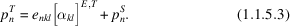International
Tables for
Crystallography
Volume D
Physical properties of crystals
Edited by A. Authier

International Tables for Crystallography (2006). Vol. D, ch. 1.1, p. 32

## Section 1.1.5.3. Relation between the pyroelectric coefficients at constant stress and at constant strain

A. Authiera*

aInstitut de Minéralogie et de la Physique des Milieux Condensés, Bâtiment 7, 140 rue de Lourmel, 75015 Paris, France
By combining relations (1.1.5.1)and (1.1.5.2), it is possible to obtain relations between the pyroelectric coefficients at constant stress,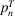, and the pyroelectric coefficients at constant strain,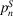, also called real pyroelectric coefficients,. Let us put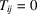and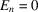in the first equation of (1.1.5.1). For a given variation of temperature,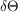, the observed strain is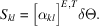From the second equations of (1.1.5.1)and (1.1.5.2), it follows that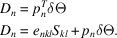Substituting the expression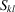and eliminating, it follows that